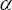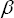# How to insert an equation with matrix

Word
This tip display how to insert an equation for example, the Electromagnetic tensor.

Do the following (how to add an equation in your document, see Working with Microsoft Equation):

1.   In the Equation Editor enter F and then select Subscript in the Subscript and superscript templates group on the Equation toolbar:2.   Chooseandin the Greek characters (lowercase) group on the Equation toolbar:3.   Enter = and then choose Parentheses, or round brackets in the Fence templates group on the Equation toolbar:4.   Choose 4x4 matrix in the Matrix templates group on the Equation toolbar:To add a matrix more than 4x4, do the following:

1. choose Variable-size matrix or table in the Matrix templates group on the Equation toolbar:2. in the Matrix dialog box enter count of columns or/and rows and other parameters what your want:5.   Enter in some positions zero, for other positions use Subscript and Full-size vertical fraction: